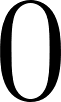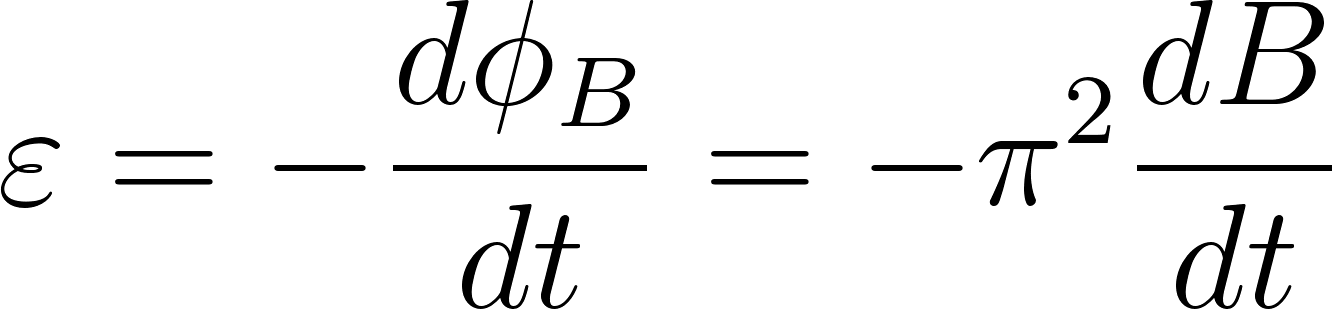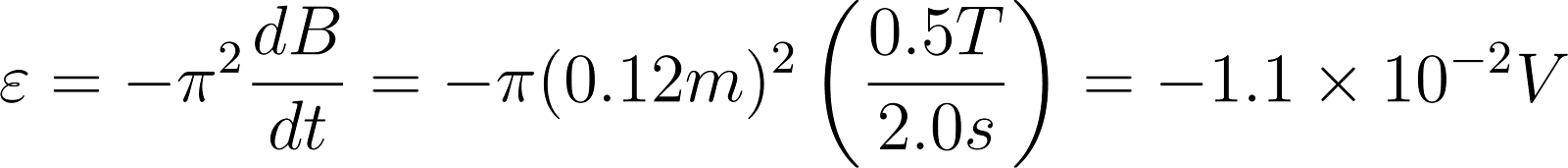×
Get Full Access to Fundamentals Of Physics - 10 Edition - Chapter 30 - Problem 4
Get Full Access to Fundamentals Of Physics - 10 Edition - Chapter 30 - Problem 4

×

# A wire loop of radius 12 cm and resistance 8.5 1 isISBN: 9781118230718 79

## Solution for problem 4 Chapter 30

Fundamentals of Physics | 10th Edition

• Textbook Solutions
• 2901 Step-by-step solutions solved by professors and subject experts
• Get 24/7 help from StudySoup virtual teaching assistantsFundamentals of Physics | 10th Edition

4 5 1 430 Reviews
13
5
Problem 4

A wire loop of radius 12 cm and resistance 8.5 1 is located in a uniform magnetic field that changes in magnitude as given in Fig. 30-35. The vertical axis scale is set by Bs ! 0.50 T, and the horizontal axis scale is set by ts ! 6.00 s. The loops plane is perpendicular to . What emf is induced in the loop during time intervals (a) 0 to 2.0 s, (b) 2.0 s to 4.0 s, and (c) 4.0 s to 6.0 s?

Step-by-Step Solution:

Problem 4

A wire loop of radius 12 cm and resistance 8.5 1 is located in a uniform magnetic field that changes in magnitude as given in figure. The vertical axis scale is set by Bs = 0.50 T, and the horizontal axis scale is set by ts = 6.00 s. The loops plane is perpendicular to . What emf is induced in the loop during time intervals (a) 0 to 2.0 s, (b) 2.0 s to 4.0 s, and (c) 4.0 s to 6.0 s?Step by Step Solution

Step 1 of 3

(a)

EMF induced in the loop during the time intervalto,use equation.ForStep 2 of 3

Step 3 of 3

##### ISBN: 9781118230718

This textbook survival guide was created for the textbook: Fundamentals of Physics, edition: 10. Since the solution to 4 from 30 chapter was answered, more than 847 students have viewed the full step-by-step answer. This full solution covers the following key subjects: axis, set, Scale, Loop, loops. This expansive textbook survival guide covers 44 chapters, and 4164 solutions. Fundamentals of Physics was written by and is associated to the ISBN: 9781118230718. The full step-by-step solution to problem: 4 from chapter: 30 was answered by , our top Physics solution expert on 09/04/17, 09:42PM. The answer to “A wire loop of radius 12 cm and resistance 8.5 1 is located in a uniform magnetic field that changes in magnitude as given in Fig. 30-35. The vertical axis scale is set by Bs ! 0.50 T, and the horizontal axis scale is set by ts ! 6.00 s. The loops plane is perpendicular to . What emf is induced in the loop during time intervals (a) 0 to 2.0 s, (b) 2.0 s to 4.0 s, and (c) 4.0 s to 6.0 s?” is broken down into a number of easy to follow steps, and 85 words.

Unlock Textbook Solution